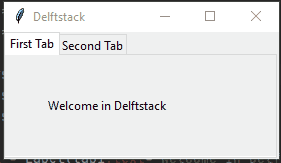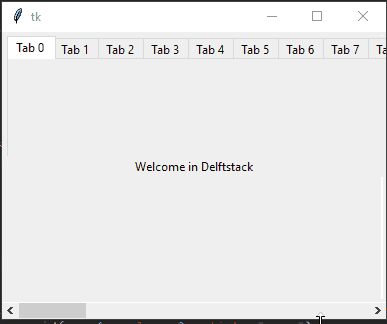# Tkinter 标签

Python 提供了一系列 GUI 框架，可以改进 Python 中基于 GUI 的应用程序。最广泛使用的 Python 接口是 `Tk` 接口或 `tkinter`

`tkinter` 模块提供了各种组件，与 Python 提供的其他链接相比，这些组件可用于更快、更轻松地升级 GUI 应用程序。`tkinter.ttk` 模块作为现有 `tk` 模块的扩展。

`Ttk` 模块加载了 18 个组件，其中 12 个在 `tkinter` 模块中，例如 `Button``Test Button``Label``Canvas``Frame``Label``Scrollbar`，等等。

## 在 Tkinter 中使用 `Notebook()` 组件创建标签

``````from tkinter import *
from tkinter import ttk
``````

Python 2 用户应该使用下面的一个。

``````from Tkinter import *
``````

``````tabsystem = ttk.Notebook(master=None, **options)
``````

`Notebook()` 采用多个参数，如下所示。

1. `master` – 此参数接受 `Tk()` 的实例。
2. `options` – 在 `Notebook` 方法中有多个选项可用于传递，例如 `height``padding``width`（这些选项是可选的）。

``````tab1 = Frame(tabsystem)
``````

`add()` 需要两个参数，如下所示。

``````add(child, **options)
``````
1. `child` – 你使用 `tabsystem` 父级由 `Frame` 类创建的 `child` 组件。在我们的例子中，`tab1` 是一个孩子。
2. `options``add()` 方法可以执行多个选项，如 `sticky``state``padding``text``image``compound``underline`
``````tabsystem.add(tab1, text='First Tab')
``````

``````tabsystem.pack(expand=1, fill="both")
``````

``````from tkinter import *
from tkinter import ttk
# Create an instance of Tk
window = Tk()
window.title("Delftstack")
# Create a tab control that manages multiple tabs
tabsystem = ttk.Notebook(window)
# Create new tabs using Frame widget
tab1 = Frame(tabsystem)
tab2 = Frame(tabsystem)
tabsystem.pack(expand=1, fill="both")
label = Label(tab1,text="Welcome in Delftstack")
label.grid(column=1,
row=1,
label2nd = Label(tab2, text="Now we are able to see another tab")
label2nd.grid(column=1,
row=1,
window.mainloop()
``````## 在 Tkinter 中使用 `Scrollbar` 组件查看多个标签

``````cnv = Canvas(window, borderwidth=1)
``````

``````frame = Frame(cnv)
``````

``````hscrollbar = Scrollbar(window, orient="horizontal", command=cnv.xview)
hscrollbar.grid(row=1, column=0, sticky="nsew")
``````

`标签` 组件也将在每个新标签中占据其位置。

``````for tab in range(40):
label = Label(tabs, text=f"Welcome in Delftstack")
label.grid(column=0,row=0, sticky="ew")
``````

``````def frame_configure(event):
global cnv
cnv.configure(scrollregion=cnv.bbox("all"))
``````

``````frame.bind("<Configure>", frame_configure)
``````

``````from tkinter import *
import tkinter.ttk as ttk
# Create a window
window = Tk()
# Create a canvas
cnv = Canvas(window, borderwidth=1)
frame = Frame(cnv)
# Create an object of horizontal scrollbar
hscrollbar = Scrollbar(window, orient="horizontal", command=cnv.xview)
hscrollbar.grid(row=1, column=0, sticky="nsew")
cnv.configure(xscrollcommand=hscrollbar.set)
cnv.grid(row=0, column=0, sticky="nsew")
# This method that helps to create canvas window
cnv.create_window((5,4), window=frame, anchor="nw", tags="frame")
tabsystem = ttk.Notebook(frame, width=100, height=100)
for tab in range(40):